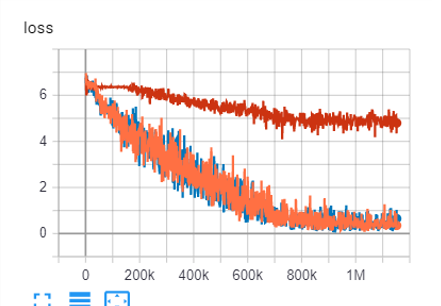# Rebuilding resnet50 from layers, doesn't work

Hi,
Im conducting research, and I would like to investigate multi branch architecture. I want to have a network that is based on resnet50, but branches based on rules at layer 4, and uses only that branch for given ‘extra_label’ input.

Im working on this for quite some time, but I cant find the reason why my architecture gives very low results, like 25-50x less perfomance. I continued striping down the architecture to find the reason for lower performance compared to simple resnet50.
In order to debug, and find the cause, I reduced my branched resnet50 architecture to just 1 branch, so this means its basicly a resnet50, rebuilt from parts, seen below.

But still, I get 25-50x less perfomance, so it has to be a bug, since its basically the same architecture.
I included some images of differences in the two architectures (copied from debug data). The left is a working good performing resnet50, the right is the result of the code above, the 1 branch architecture, named PABN.

``````class PABN(nn.Module):
def __init__(self):
super(PABN, self).__init__()
num_classes = 576

self.backbone = list(resnet50(pretrained=True).children())[:-3]  # removes conv 5, avgpool, fully con
self.backbone = nn.Sequential(*self.backbone)

self.branches = nn.ModuleList()
[self.branches.append(self._get_shared_branch_backbone()) for _ in range(1)]

self.fcs = nn.ModuleList()
[self.fcs.append(nn.Linear(2048, num_classes)) for _ in range(1)]

def _get_shared_branch_backbone():
net = list(resnet50(pretrained=True).children())[7:-1]  # Gets conv5 + AAP2
return nn.Sequential(*net)

def forward(self, images, extra_label):
batch_size=images.size()
# forward shared backbone:
x_backbone_out = self.backbone(images)

# forward of separate branch based on extra_label label
branch_outs = []
for inp, e_l in zip(x_backbone_out, extra_label):
e_l=e_l.item() # to get an integer for indexing
inp_exp=torch.unsqueeze(inp, 0)  # add the batch dim
x = self.branches[e_l](inp_exp)
x = x.squeeze(3).squeeze(2)
branch_outs.append(x)
branch_outs = torch.cat(branch_outs, 0)

if not self.training:  # so IF TESTING-eval, return the feature vector:
return branch_outs

branch_outs_fc = []
for inp, e_l in  zip(branch_outs, extra_label):  # use the fully connected layers
e_l=e_l.item()
inp_exp = torch.unsqueeze(inp, 0)  # add the batch dim
branch_outs_fc.append(self.fcs[e_l](inp_exp))
branch_outs_fc = torch.cat(branch_outs_fc, 0)
return branch_outs_fc``````

What does performance refer to in this context?
The end to end throught (e.g. samples per second or training time for an epoch) or model accuracy?

I meant performance as model accuracy. I use mAP as performance measure. The goal is to conclude on the similarity of inputs, that is why I have an early return of feature vector in the if not self.training: return branch_outs. During training it can be looked at as a simple classification problem

Also loss does not go down during training:The orange/blue line is a good performing resnet50, the red is my own rebuilt model.

Also note that every other part of my code is the same, such as loss, optimizer, dataloader, data, etc. I investigated thoroughly and im pretty sure the problem is the model file.

The code will output the same result as the reptrained resnet50 during evaluation (and using the same `self.fc` at the end for the sake of debugging).
Code snippet:

``````class PABN(nn.Module):
def __init__(self):
super(PABN, self).__init__()
num_classes = 576

self.backbone = list(models.resnet50(pretrained=True).children())[:-3]  # removes conv 5, avgpool, fully con
self.backbone = nn.Sequential(*self.backbone)

self.branches = nn.ModuleList()
[self.branches.append(self._get_shared_branch_backbone()) for _ in range(1)]

self.fcs = nn.ModuleList()
[self.fcs.append(list(models.resnet50(pretrained=True).children())[-1]) for _ in range(1)]

def _get_shared_branch_backbone(self):
net = list(models.resnet50(pretrained=True).children())[7:-1]  # Gets conv5 + AAP2
return nn.Sequential(*net)

def forward(self, images, extra_label):
batch_size=images.size()
# forward shared backbone:
x_backbone_out = self.backbone(images)

# forward of separate branch based on extra_label label
branch_outs = []
for inp, e_l in zip(x_backbone_out, extra_label):
e_l=e_l.item() # to get an integer for indexing
inp_exp=torch.unsqueeze(inp, 0)  # add the batch dim
x = self.branches[e_l](inp_exp)
x = x.squeeze(3).squeeze(2)
branch_outs.append(x)
branch_outs = torch.cat(branch_outs, 0)

#if not self.training:  # so IF TESTING-eval, return the feature vector:
#    return branch_outs

branch_outs_fc = []
for inp, e_l in  zip(branch_outs, extra_label):  # use the fully connected layers
e_l=e_l.item()
inp_exp = torch.unsqueeze(inp, 0)  # add the batch dim
branch_outs_fc.append(self.fcs[e_l](inp_exp))
branch_outs_fc = torch.cat(branch_outs_fc, 0)
return branch_outs_fc

model = PABN().eval()
x = torch.randn(2, 3, 224, 224)
output = model(x, torch.tensor([0, 0]))

model_reference = models.resnet50(pretrained=True).eval()
output_reference = model_reference(x)

print((output - output_reference).abs().max())